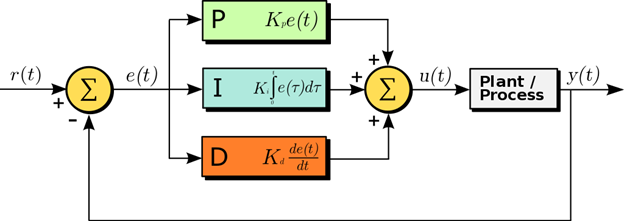Home » »

# Red Pitaya as a PID controllerA proportional-integral-derivative controller is a control loop mechanism often used in industrial control systems where continuous control is needed. The easiest way to understand one is by breaking it down to individual elements (proportional (P), integral (I) and derivative (D)), as can be seen in the diagram above.

The PID control algorithm calculates the difference e(t) between the setpoint r(t) and process variable y(t) and feeds it into the following three functions:

• To calculate the proportional gain, e(t) is simply multiplied with a set constant Kp. This means that if the setpoint is significantly above the process variable then the proportional gain will be large and positive.
• With the integral gain we account for the past values of e(t), meaning that the integral gain will be positive as long as the sum of all errors in the observed interval is positive. This term can be zero if the process value is equal to the setpoint across the entire interval, or if it is oscillating around the setpoint in a way that the errors cancel each other out.
• The derivative gain depends on how fast e(t) is changing. If e(t) is positive and decreasing rapidly, the gain will be negative and large. With this we try to avoid overshooting the setpoint.

In order for a PID controller to serve its purpose, it also needs to be tuned. This means adjusting the algorithm parameters (Kp, Kd, Ki and integrator reset) to achieve the desired control response, which will be different from application to application, and may even have conflicting requirements. There are many tuning techniques that are available there from simply tuning the system manually (which requires experience), through heuristic tuning methods such as the Ziegler-Nichols method and sophisticated software solutions.

Red Pitaya PID controller

Among other applications, Red Pitaya can also be used as a PID controller. Its MIMO PID controller consists of 4 standard PID controllers with P, I, and D parameter settings and integrator reset control. The PID can be controlled through FPGA registers that are described inside the PID controller section of the FPGA register map. This makes Red Pitaya a very fast and capable PID controller.

Of course, as a true Swiss Army knife, working as a PID controller is just one of the many things a Red Pitaya can do. To see what else is possible with it, click here.